# CAT 2017 – Slot 1 – Logical Reasoning and Data Interpretation – Simple Happiness index (SHI) of a country is computed on the basis of three parameters: social support (S), freedom to life choices (F) and corruption perception (C)

##### Simple Happiness index (SHI) of a country is computed on the basis of three parameters: social support (S), freedom to life choices (F) and corruption perception (C). Each of these three parameters is measured on a scale of 0 to 8 (integers only). A country is then categorized based on the total score obtained by summing the scores of ail the three parameters, as shown in the following table: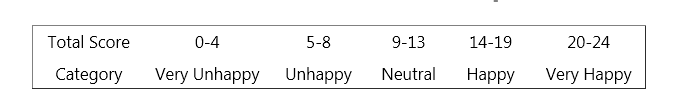Following diagram depicts the frequency distribution of the scores in S, F and C of 10 countries - Amda, Benga, Calla, Delma, Eppa, Varsa, Wanna, Xanda, Yanga and Zoorna;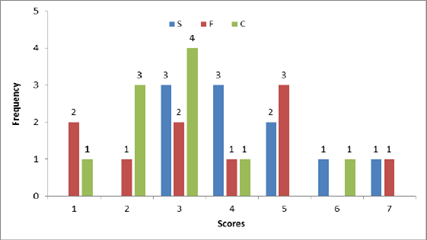Further, the following are known: 1. Amda and Calls jointly have the lowest total score, 7, with identical scores in all the three parameters. 2. Zooma has a total score of 17. 3. All the 3 countries, which are categorised as happy, have the highest score in exactly one parameter.

Q. 1: What is Amda’s score in F?

##### Q. 2: What is Zooma’s score in S?

Q. 3: Benga and Delma, two countries categorized as happy, are tied with the same total score. What is the maximum score they can have?
A) 14 B) 15 C) 16 D) 17

Q. 4: If Benga scores 16 and Delma scores 15, then what is the maximum number of countries with a score of 13?
A) 0 B) 1 C) 2 D) 3

Explanation:- The given data can be represented in a table as follows.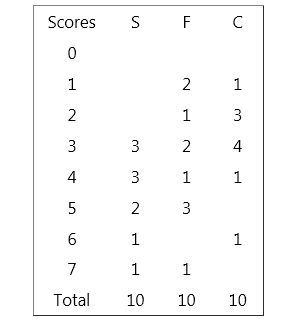A and C had a total score of 7, with identical scores in all these parameters. So it can only be 1, 2 and 4 or 3, 3 and 1. As Zooma    has a score of 17, and all three countries in the happy category had the highest score in exactly one parameter, he can only have a   7 in F, 6 in S and 4 in C as a score of 7 in S and 6 in C would be the scores of the other two countries and he cannot have a 7, 7    and 5 as there is no country which scored a 5 in C.

Amda can have a distribution of 3, 3, 1 or 4, 2, 1. In either case the only possible score of F is 1 as no other parameter has a score    of 1 for two countries.

A and C had a total score of 7, with identical scores in all these parameters. So it can only be 1, 2 and 4 or 3, 3 and 1. As Zooma    has a score of 17, and all three countries in the happy category had the highest score in exactly one parameter, he can only have a   7 in F, 6 in S and 4 in C as a score of 7 in S and 6 in C would be the scores of the other two countries and he cannot have a 7, 7    and 5 as there is no country which scored a 5 in C.

As explained before Zooma’s score in C has to be 6.

Explanation:- The given data can be represented in a table as follows.
A and C had a total score of 7, with identical scores in all these parameters. So it can only be 1, 2 and 4 or 3, 3 and 1. As Zooma   has a score of 17, and all three countries in the happy category had the highest score in exactly one parameter, he can only have a  7 in F, 6 in S and 4 in C as a score of 7 in S and 6 in C would be the scores of the other two countries and he cannot have a 7, 7    and 5 as there is no country which scored a 5 in C.

In the table given, among the highest scores, a score of 7 in F, 6 in S and 4 in S were the score of Zoom. The best possible scores remaining for Benga and Dalma would be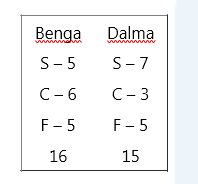As it is given that both had the some total score it can only be 15 for both , i.e. Benga’s score in S or F was one less than the maximum possible.

A and C had a total score of 7, with identical scores in all these parameters. So it can only be 1, 2 and 4 or 3, 3 and 1. As Zooma   has a score of 17, and all three countries in the happy category had the highest score in exactly one parameter, he can only have a  7 in F, 6 in S and 4 in C as a score of 7 in S and 6 in C would be the scores of the other two countries and he cannot have a 7, 7    and 5 as there is no country which scored a 5 in C.

Considering the score of Zoom, Benga and Delma as 17, 16 and 15, we get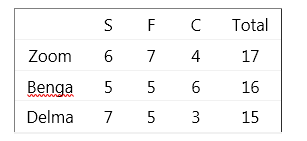If Benga score 16 and Dalma score 15 (as illustrated in the previous solution) the maximum possible values remaining are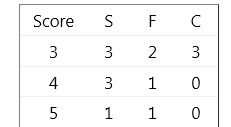##### Checkout Other Questions of CAT 2017 Slot 1 Paper:

Verbal Ability :              |   Q.01- Q.06  |  Q.07- Q.12  |  Q.13- Q.18  |  Q.19- Q.21  |  Q.22- Q.24  |  Q.25- Q.29  |  Q.30 – Q.34  |

Logical Reasoning :    |   Q.29 – Q.32  |

Quantitative Aptitude: |

### Free Material Area